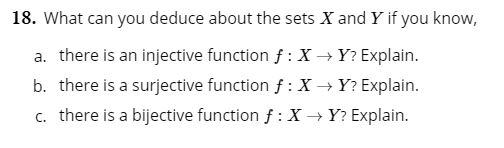# 18. What can you deduce about the sets X and Y if you know,a. there is an injective function f: X Y? Explain.b. there is a surjective functionf:XY? Explain.c. there is a bijective function f: XY? Explain.

Question
16 viewshelp_outlineImage Transcriptionclose18. What can you deduce about the sets X and Y if you know, a. there is an injective function f: X Y? Explain. b. there is a surjective functionf:X Y? Explain. c. there is a bijective function f: XY? Explain. fullscreen
check_circle

star
star
star
star
star
1 Rating
Step 1

Given that two sets X and Y.

a)There is an injective function

Step 2

This means

Step 3

That is

f(a) = f(s)

this implies that

a = s

b)There ...

### Want to see the full answer?

See Solution

#### Want to see this answer and more?

Solutions are written by subject experts who are available 24/7. Questions are typically answered within 1 hour.*

See Solution
*Response times may vary by subject and question.
Tagged in